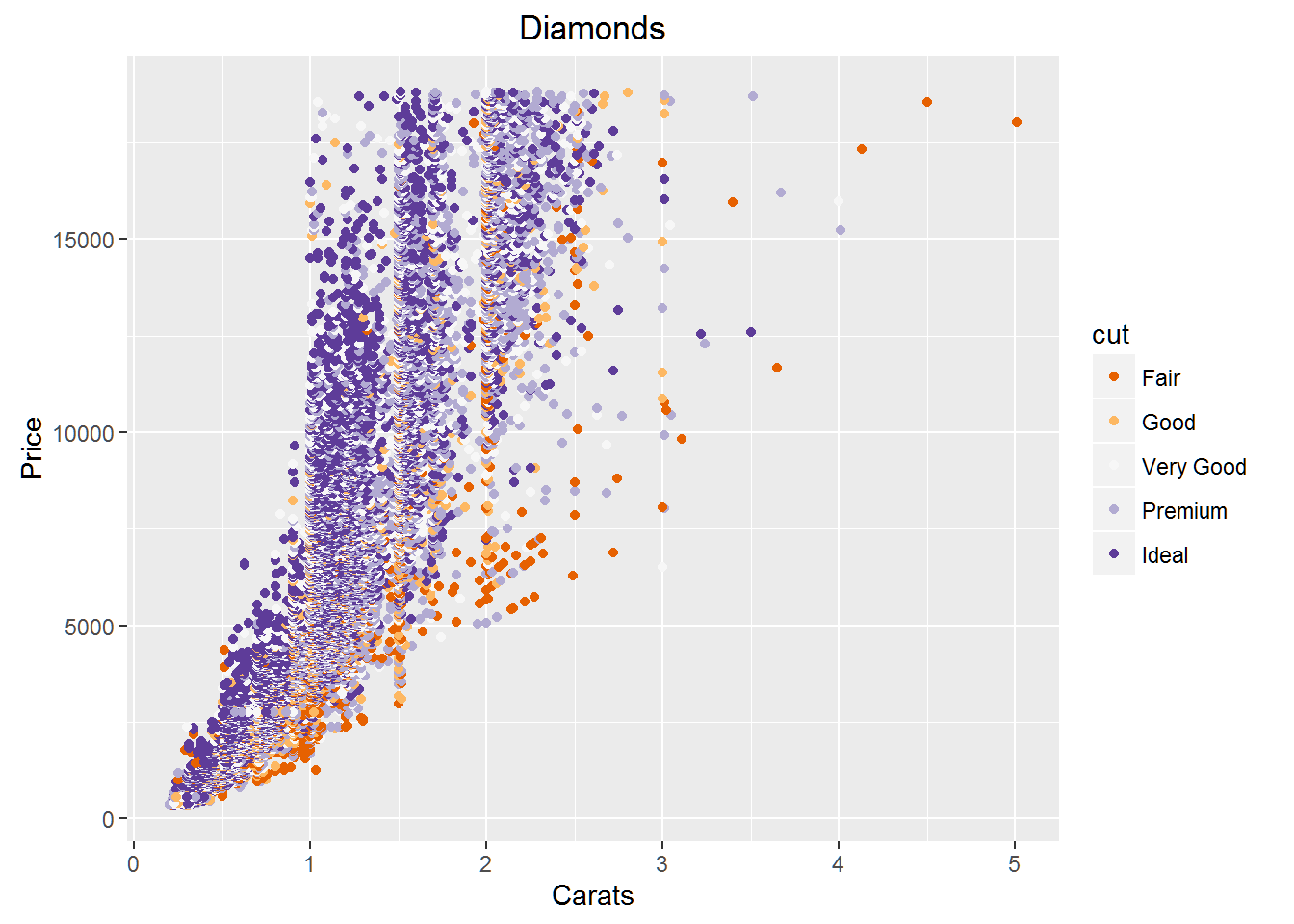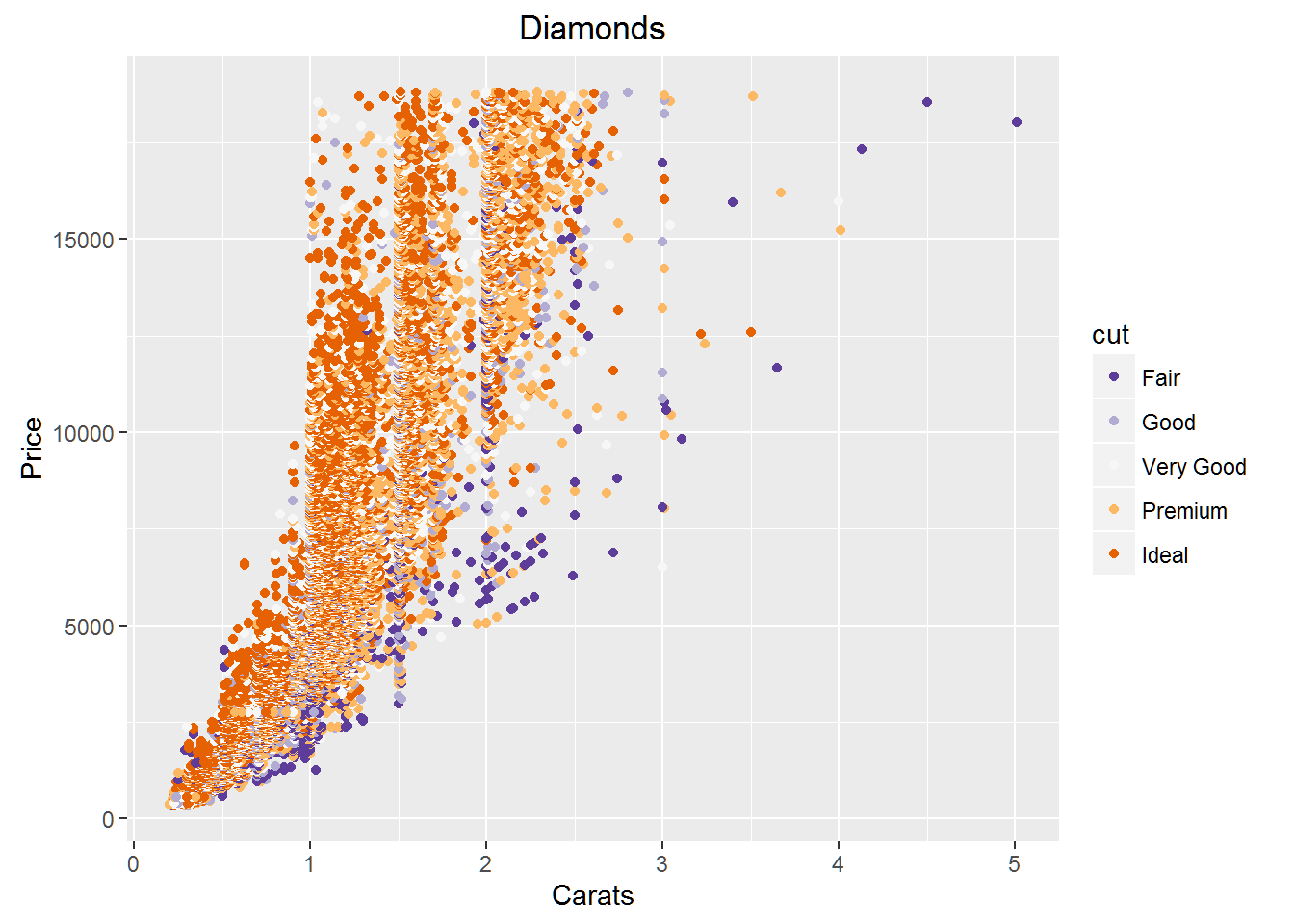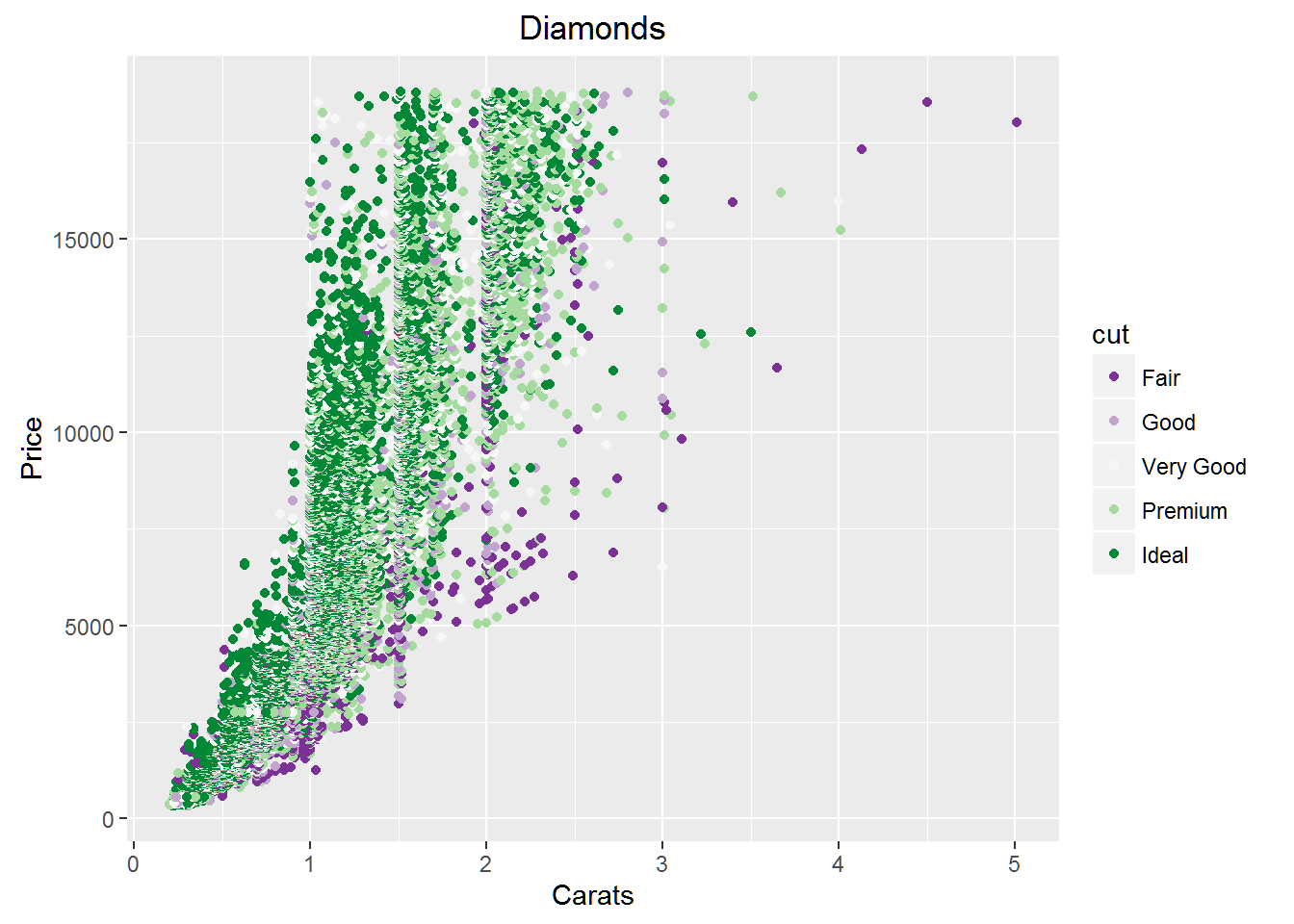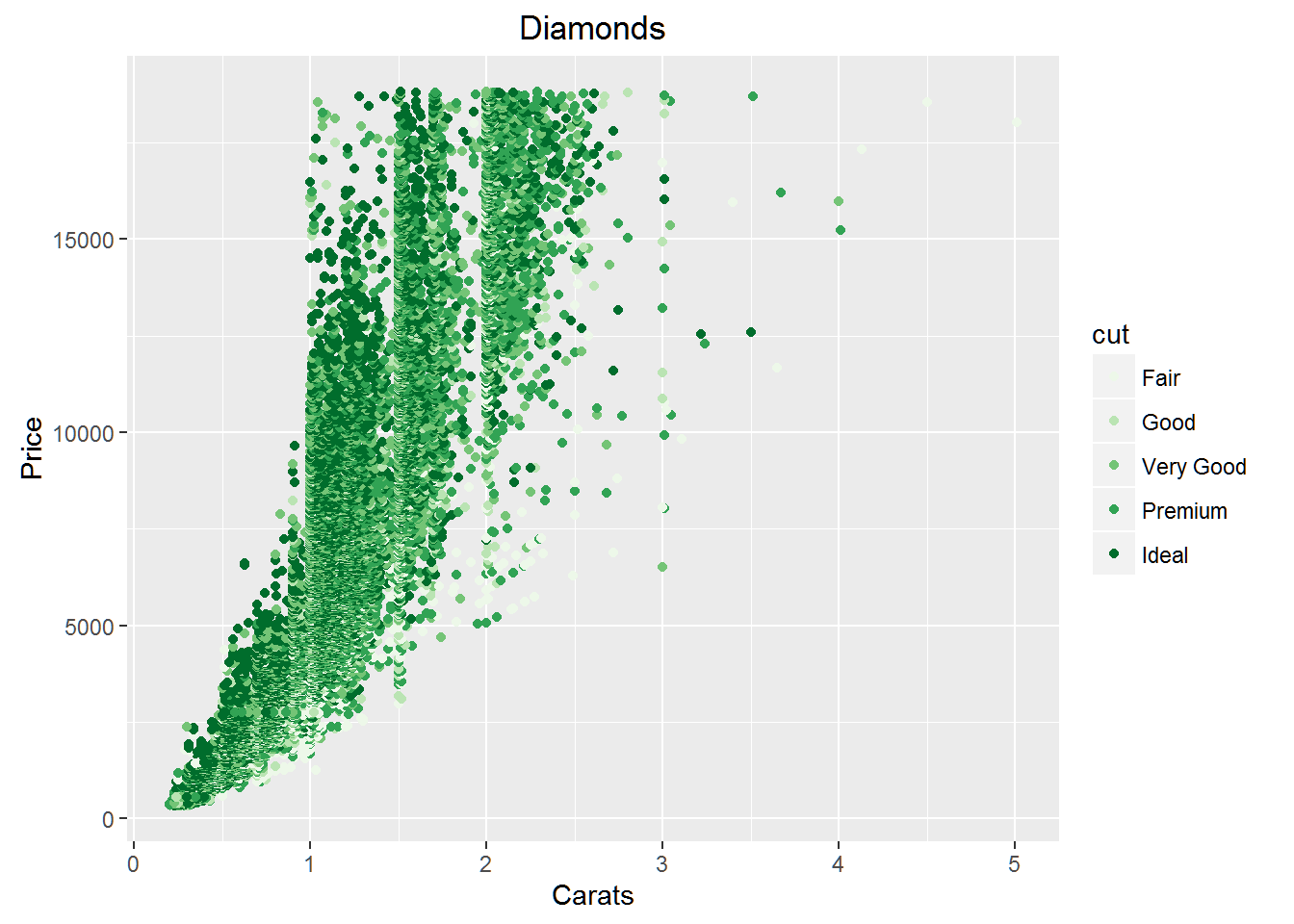A great feature of R is its graphic capability. The ggplot package is well-known for its powerful charting kit, which features plenty of color options.

The color brewer color scale includes over 250 palettes. This makes for a lot of choices when creating chart graphics in R.

We’re going to look at a single chart of data from R’s Diamonds data set and explore different color schemes. More information on these palettes can be found at colorbrewer2.org You can also download the color chart in Excel form here on the site.

We going to find the difficult part isn’t creating a color scheme that suits our design or graphic purposes but selecting one from the many options found in color brewer.

``library(ggplot2)``

We can use the ggplot function to create a basic x-y scatterplot of data from the Diamonds set to convey three levels of information. We will plot diamonds by carat and price, and we’ll use cut to classify the data points.

The flexibility of ggplot2 is well displayed here. In our first chart, we use scale_color_brewer to specify the color scheme we want to use, and we will label the chart axes and give it a title.

``ggplot(diamonds, aes(carat, price)) + geom_point(aes(color = cut)) + scale_color_brewer(type = 'div', palette = 4, direction = 1) + xlab('Carats') + ylab('Price') + ggtitle('Diamonds')``In this next chart we will make just two changes to show how we can get the same results with some alterations to the code. This time, we specify color = cut in the first usage of aes(). We will also reverse the direction of the colors by indicating -1 rather than the original 1 setting.

``ggplot(diamonds, aes(carat, price, color = cut)) + geom_point() + scale_color_brewer(type = 'div', palette = 4, direction = -1) + xlab('Carats') + ylab('Price') + ggtitle('Diamonds')``From this point on, it’s just variations on a theme. All we are trying to do is experiment with different color settings to find one best suited to our purposes.

We can specify a palette by using its code, PRGn in this case.

``ggplot(diamonds, aes(carat, price, color = cut)) + geom_point() + scale_color_brewer(type = 'div', palette = 'PRGn', direction = 1) + xlab('Carats') + ylab('Price') + ggtitle('Diamonds')``Or we can specify a color group. Five follow: Greens, Reds, Blues, Oranges, and Greys.

``ggplot(diamonds, aes(carat, price, color = cut)) + geom_point() + scale_color_brewer(type = 'div', palette = 'Greens', direction = 1) + xlab('Carats') + ylab('Price') + ggtitle('Diamonds')````ggplot(diamonds, aes(carat, price, color = cut)) + geom_point() + scale_color_brewer(type = 'div', palette = 'Reds', direction = 1) + xlab('Carats') + ylab('Price') + ggtitle('Diamonds')``